# Getting Started with PyTorch

• Last Updated : 17 May, 2022

Deep Learning is a branch of Machine Learning where algorithms are written which mimic the functioning of a human brain. The most commonly used libraries in deep learning are Tensorflow and PyTorch. As there are various deep learning frameworks available, one might wonder when to use PyTorch. Here are reasons why one might prefer using Pytorch for specific tasks.

Pytorch is an open-source deep learning framework available with a Python and C++ interface. Pytorch resides inside the torch module. In PyTorch, the data that has to be processed is input in the form of a tensor.

## Installing PyTorch

If you have Anaconda Python Package manager installed in your system, then using by running the following command in the terminal will install PyTorch:

conda install pytorch torchvision cpuonly -c pytorch

If you want to use PyTorch without explicitly installing it into your local machine, you can use Google Colab.

## PyTorch tensors

The Pytorch is used to process the tensors. Tensors are multidimensional arrays like n-dimensional NumPy array. However, tensors can be used in GPUs as well, which is not in the case of NumPy array. PyTorch accelerates the scientific computation of tensors as it has various inbuilt functions.

A vector is a one-dimensional tensor, and a matrix is a two-dimensional tensor. One significant difference between the Tensor and multidimensional array used in C, C++, and Java is tensors should have the same size of columns in all dimensions. Also, the tensors can contain only numeric data types.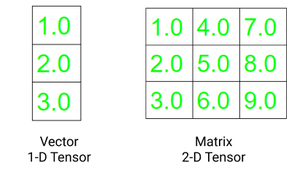The two fundamental attributes of a tensor are:

• Shape: refers to the dimensionality of array or matrix
• Rank: refers to the number of dimensions present in tensor

Code:

## Python3

 `# importing torch` `import` `torch`   `# creating a tensors` `t1``=``torch.tensor([``1``, ``2``, ``3``, ``4``])` `t2``=``torch.tensor([[``1``, ``2``, ``3``, ``4``],` `                 ``[``5``, ``6``, ``7``, ``8``],` `                 ``[``9``, ``10``, ``11``, ``12``]])`   `# printing the tensors:` `print``(``"Tensor t1: \n"``, t1)` `print``(``"\nTensor t2: \n"``, t2)`   `# rank of tensors` `print``(``"\nRank of t1: "``, ``len``(t1.shape))` `print``(``"Rank of t2: "``, ``len``(t2.shape))`   `# shape of tensors` `print``(``"\nRank of t1: "``, t1.shape)` `print``(``"Rank of t2: "``, t2.shape)`

Output: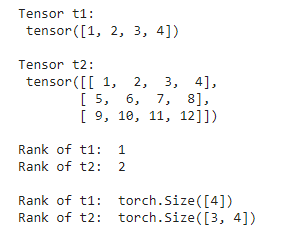## Creating Tensor in PyTorch

There are various methods to create a tensor in PyTorch.  A tensor can contain elements of a single data type. We can create a tensor using a python list or NumPy array. The torch has 10 variants of tensors for both GPU and CPU. Below are different ways of defining a tensor.

torch.Tensor() : It copies the data and creates its tensor. It is an alias for torch.FloatTensor.

torch.tensor() : It also copies the data to create a tensor; however, it infers the data type automatically.

torch.as_tensor() : The data is shared and not copied in this case while creating the data and accepts any type of array for tensor creation.

torch.from_numpy() : It is similar to tensor.as_tensor() however it accepts only numpy array.

Code:

## Python3

 `# importing torch module` `import` `torch` `import` `numpy as np`   `# list of values to be stored as tensor` `data1 ``=` `[``1``, ``2``, ``3``, ``4``, ``5``, ``6``]` `data2 ``=` `np.array([``1.5``, ``3.4``, ``6.8``,` `                ``9.3``, ``7.0``, ``2.8``])`   `# creating tensors and printing ` `t1 ``=` `torch.tensor(data1)` `t2 ``=` `torch.Tensor(data1)` `t3 ``=` `torch.as_tensor(data2)` `t4 ``=` `torch.from_numpy(data2)`   `print``(``"Tensor: "``,t1, ``"Data type: "``, t1.dtype,``"\n"``)` `print``(``"Tensor: "``,t2, ``"Data type: "``, t2.dtype,``"\n"``)` `print``(``"Tensor: "``,t3, ``"Data type: "``, t3.dtype,``"\n"``)` `print``(``"Tensor: "``,t4, ``"Data type: "``, t4.dtype,``"\n"``)`

Output: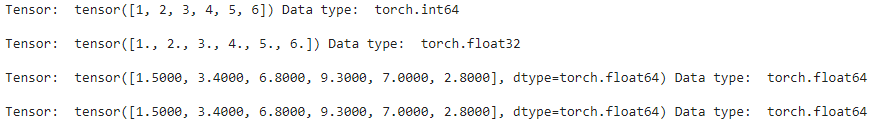## Restructuring Tensors in Pytorch

We can modify the shape and size of a tensor as desired in PyTorch. We can also create a transpose of an n-d tensor. Below are three common ways to change the structure of your tensor as desired:

.reshape(a, b) : returns a new tensor with size a,b

.resize(a, b) : returns the same tensor with the size a,b

.transpose(a, b) : returns a tensor transposed in a and b dimension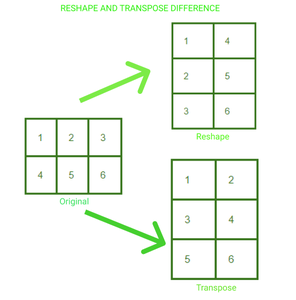A 2*3 matrix has been reshaped and transposed to 3*2. We can visualize the change in the arrangement of the elements in the tensor in both cases.

Code:

## Python3

 `# import torch module` `import` `torch `   `# defining tensor` `t ``=` `torch.tensor([[``1``, ``2``, ``3``, ``4``],` `                 ``[``5``, ``6``, ``7``, ``8``],` `                 ``[``9``, ``10``, ``11``, ``12``]])`   `# reshaping the tensor` `print``(``"Reshaping"``)` `print``(t.reshape(``6``, ``2``))`   `# resizing the tensor` `print``(``"\nResizing"``)` `print``(t.resize(``2``, ``6``))`   `# transposing the tensor` `print``(``"\nTransposing"``)` `print``(t.transpose(``1``, ``0``))`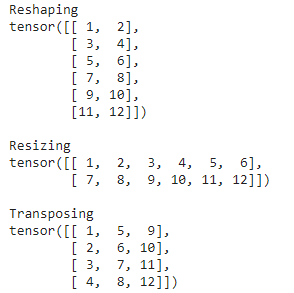## Mathematical Operations on Tensors in PyTorch

We can perform various mathematical operations on tensors using Pytorch. The code for performing Mathematical operations is the same as in the case with NumPy arrays. Below is the code for performing the four basic operations in tensors.

## Python3

 `# import torch module` `import` `torch `   `# defining two tensors` `t1 ``=` `torch.tensor([``1``, ``2``, ``3``, ``4``])` `t2 ``=` `torch.tensor([``5``, ``6``, ``7``, ``8``])`   `# adding two tensors` `print``(``"tensor2 + tensor1"``)` `print``(torch.add(t2, t1))`   `# subtracting two tensor` `print``(``"\ntensor2 - tensor1"``)` `print``(torch.sub(t2, t1))`   `# multiplying two tensors` `print``(``"\ntensor2 * tensor1"``)` `print``(torch.mul(t2, t1))`   `# diving two tensors` `print``(``"\ntensor2 / tensor1"``)` `print``(torch.div(t2, t1))`

Output: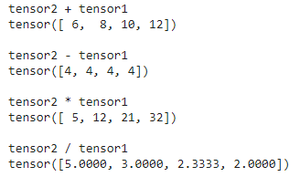For getting into further in-depth matrix multiplication using Pytorch please refer to this article.

## Pytorch Modules

The PyTorch library modules are essential to create and train neural networks. The three main library modules are Autograd, Optim, and nn.

# 1. Autograd Module: The autograd provides the functionality of easy calculation of gradients without the explicitly manual implementation of forward and backward pass for all layers.

For training any neural network we perform backpropagation to calculate the gradient. By calling the .backward() function we can calculate every gradient from the root to the leaf.

Code:

## Python3

 `# importing torch` `import` `torch`   `# creating a tensor` `t1``=``torch.tensor(``1.0``, requires_grad ``=` `True``)` `t2``=``torch.tensor(``2.0``, requires_grad ``=` `True``)`   `# creating a variable and gradient` `z``=``100` `*` `t1 ``*` `t2` `z.backward()`   `# printing gradient` `print``(``"dz/dt1 : "``, t1.grad.data)` `print``(``"dz/dt2 : "``, t2.grad.data)`

Output: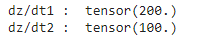# 2. Optim Module: PyTorch Optium Module which helps in the implementation of various optimization algorithms. This package contains the most commonly used algorithms like Adam, SGD, and RMS-Prop. To use torch.optim we first need to construct an Optimizer object which will keep the parameters and update it accordingly. First, we define the Optimizer by providing the optimizer algorithm we want to use. We set the gradients to zero before backpropagation. Then for updation of parameters the optimizer.step() is called.

optimizer = torch.optim.Adam(model.parameters(), lr=0.01) #defining optimizer

optimizer.step() #parameter updation

# 3. nn Module: This package helps in the construction of neural networks. It is used to build layers.

For creating a model with a single layer we can simply define it by using nn.Sequential().

model = nn.Sequential( nn.Linear(in, out), nn.Sigmoid(), nn.Linear(_in, _out), nn.Sigmoid() )

For the implementation of the model which is not in a single sequence, we define a model by subclassing the nn.Module class.

## Python3

 `class` `Model (nn.Module) : `   `         ``def` `__init__(``self``): `   `              ``super``(Model, ``self``).__init__()`   `              ``self``.linear ``=` `torch.nn.Linear(``1``, ``1``)  `   `      ``def` `forward(``self``, x):`   `              ``y_pred ``=` `self``.linear(x)`   `             ``return` `y_pred`

## PyTorch Dataset and Dataloader

The torch.utils.data.Dataset class contains all the custom datasets. We need to implement two methods, __len__() and __get_item__(), to create our own dataset class.

The PyTorch Dataloader has an amazing feature of loading the dataset in parallel with automatic batching. It, therefore, reduces the time of loading the dataset sequentially hence enhancing the speed.

Syntax: DataLoader(dataset, shuffle=True, sampler=None, batch_sampler=None, batch_size=32)

The PyTorch DataLoader supports two types of datasets:

• Map-style datasets: The data items are mapped to the indexes. In these datasets, the __get_item__() method is used to retrieve the index of each item.
• Iterable-style datasets: In these datasets __iter__() protocol is implemented. The data samples are retrieved in sequence.

Please refer to the article on Using a DataLoader in PyTorch to know more.

## Building Neural Network with PyTorch

We will see this in a stepwise implementation:

1. Dataset Preparation: As everything in PyTorch is represented in the form of tensors, so we should first in tensors.
2. Building model: For building a neutral network first we first define the number of input layers, hidden layers, and output layers. We also need to define the initial weights. The values of the weights matrices are chosen randomly using torch.randn(). Torch.randn() returns a tensor consisting of random numbers from a standard normal distribution.
3. Forward Propagation: The data is fed to a neural network and a matrix multiplication is performed between the weights and input. This can be easily done using a torch.
4. Loss computation: PyTorch.nn functions have multiple loss functions. Loss functions are used to measure the error between the predicted value to the target value.
5. Backpropagation: It is used for optimizing weights. The weights are changed such that the loss is minimized.

Now let’s build a neural network from scratch:

## Python3

 `# importing torch` `import` `torch`   `# training input(X) and output(y)` `X ``=` `torch.Tensor([[``1``], [``2``], [``3``],` `                ``[``4``], [``5``], [``6``]])` `y ``=` `torch.Tensor([[``5``], [``10``], [``15``],` `                  ``[``20``], [``25``], [``30``]])`   `class` `Model(torch.nn.Module):`   `    ``# defining layer` `    ``def` `__init__(``self``):` `        ``super``(Model, ``self``).__init__()` `        ``self``.linear ``=` `torch.nn.Linear(``1``, ``1``) ` `    `  `    ``# implementing forward pass` `    ``def` `forward(``self``, x):` `        ``y_pred ``=` `self``.linear(x)` `        ``return` `y_pred`   `model ``=` `torch.nn.Linear(``1` `, ``1``)`   `# defining loss function and optimizer` `loss_fn ``=` `torch.nn.L1Loss()` `optimizer ``=` `torch.optim.Adam(model.parameters(), lr ``=` `0.01` `)`   `for` `epoch ``in` `range``(``1000``):` `   `  `    ``# predicting y using initial weights` `    ``y_pred ``=` `model(X.requires_grad_())`   `    ``# loss calculation` `    ``loss ``=` `loss_fn(y_pred, y)`   `    ``# calculating gradients` `    ``loss.backward()`   `    ``# updating weights` `    ``optimizer.step()`   `    ``optimizer.zero_grad()`   `# testing on new data    ` `X ``=` `torch.Tensor([[``7``], [``8``]])` `predicted ``=` `model(X)` `print``(predicted)`

Output: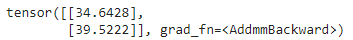My Personal Notes arrow_drop_up
Recommended Articles
Page :